Next: Perihelion precession of Mercury Up: Useful mathematics Previous: Matrix eigenvalue theory

# Spherical harmonics

The spherical harmonics, denoted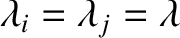, where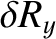is a non-negative integer, andan integer lying in the range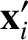, are the well-behaved solutions to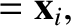(A.159)

on the surface of a sphere (i.e.,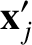constant). Here,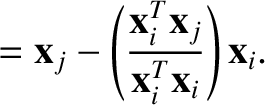denotes a Laplacian (Riley 1974a), and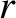,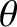,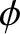are standard spherical coordinates. The spherical harmonics take the form (Jackson 1975)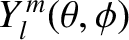(A.160)

where the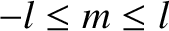are associated Legendre polynomials (Abramowitz and Stegun 1965a). In particular,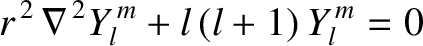(A.161)

where the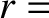are the Legendre polynomials introduced in Section 3.4. The spherical harmonics satisfy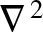(A.162)

and have the property that they are orthonormal when integrated over the surface of a sphere; that is,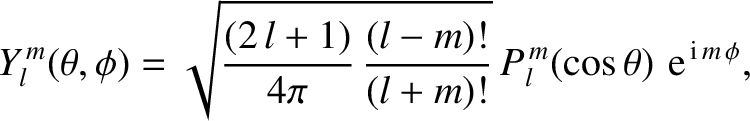(A.163)

The first few spherical harmonics are: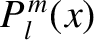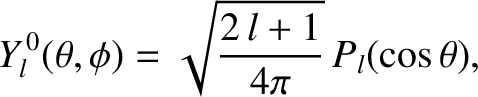(A.164)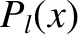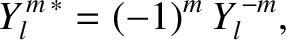(A.165)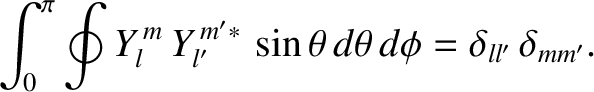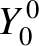(A.166)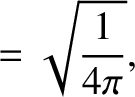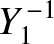(A.167)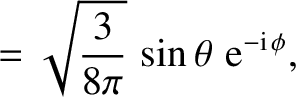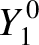(A.168)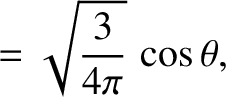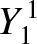(A.169)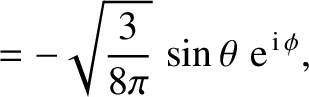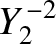(A.170)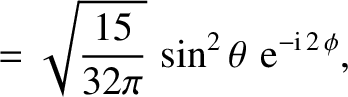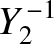(A.171) and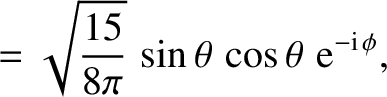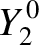(A.172)Next: Perihelion precession of Mercury Up: Useful mathematics Previous: Matrix eigenvalue theory
Richard Fitzpatrick 2016-03-31